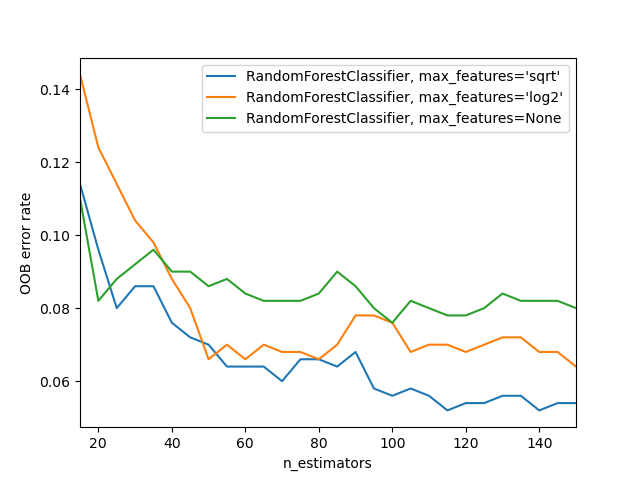# OOB Errors for Random Forests¶

The RandomForestClassifier is trained using bootstrap aggregation, where each new tree is fit from a bootstrap sample of the training observations $$z_i = (x_i, y_i)$$. The out-of-bag (OOB) error is the average error for each $$z_i$$ calculated using predictions from the trees that do not contain $$z_i$$ in their respective bootstrap sample. This allows the RandomForestClassifier to be fit and validated whilst being trained .

The example below demonstrates how the OOB error can be measured at the addition of each new tree during training. The resulting plot allows a practitioner to approximate a suitable value of n_estimators at which the error stabilizes.# Author: Kian Ho <hui.kian.ho@gmail.com>
#         Gilles Louppe <g.louppe@gmail.com>
#         Andreas Mueller <amueller@ais.uni-bonn.de>
#

from collections import OrderedDict

import matplotlib.pyplot as plt

from sklearn.datasets import make_classification
from sklearn.ensemble import RandomForestClassifier

RANDOM_STATE = 123

# Generate a binary classification dataset.
X, y = make_classification(
n_samples=500,
n_features=25,
n_clusters_per_class=1,
n_informative=15,
random_state=RANDOM_STATE,
)

# NOTE: Setting the warm_start construction parameter to True disables
# support for parallelized ensembles but is necessary for tracking the OOB
# error trajectory during training.
ensemble_clfs = [
(
"RandomForestClassifier, max_features='sqrt'",
RandomForestClassifier(
warm_start=True,
oob_score=True,
max_features="sqrt",
random_state=RANDOM_STATE,
),
),
(
"RandomForestClassifier, max_features='log2'",
RandomForestClassifier(
warm_start=True,
max_features="log2",
oob_score=True,
random_state=RANDOM_STATE,
),
),
(
"RandomForestClassifier, max_features=None",
RandomForestClassifier(
warm_start=True,
max_features=None,
oob_score=True,
random_state=RANDOM_STATE,
),
),
]

# Map a classifier name to a list of (<n_estimators>, <error rate>) pairs.
error_rate = OrderedDict((label, []) for label, _ in ensemble_clfs)

# Range of n_estimators values to explore.
min_estimators = 15
max_estimators = 150

for label, clf in ensemble_clfs:
for i in range(min_estimators, max_estimators + 1, 5):
clf.set_params(n_estimators=i)
clf.fit(X, y)

# Record the OOB error for each n_estimators=i setting.
oob_error = 1 - clf.oob_score_
error_rate[label].append((i, oob_error))

# Generate the "OOB error rate" vs. "n_estimators" plot.
for label, clf_err in error_rate.items():
xs, ys = zip(*clf_err)
plt.plot(xs, ys, label=label)

plt.xlim(min_estimators, max_estimators)
plt.xlabel("n_estimators")
plt.ylabel("OOB error rate")
plt.legend(loc="upper right")
plt.show()


Total running time of the script: (0 minutes 3.878 seconds)

Gallery generated by Sphinx-Gallery